xStoichiometryEncyclopedia
Stoichiometry is a branch of chemistry
Chemistry
Chemistry is the science of matter, especially its chemical reactions, but also its composition, structure and properties. Chemistry is concerned with atoms and their interactions with other atoms, and particularly with the properties of chemical bonds....

that deals with the relative quantities of reactants and products
Product (chemistry)
Product are formed during chemical reactions as reagents are consumed. Products have lower energy than the reagents and are produced during the reaction according to the second law of thermodynamics. The released energy comes from changes in chemical bonds between atoms in reagent molecules and...

in chemical reactions. In a balanced chemical reaction, the relations among quantities of reactants and products typically form a ratio of whole numbers. For example, in a reaction that forms ammonia (NH3), exactly one molecule of nitrogen (N2) reacts with three molecules of hydrogen (H2) to produce two molecules of NH3:
N2 + 3H2 → 2NH3

Stoichiometry can be used to calculate quantities such as the amount of products (in mass, moles, volume, etc.) that can be produced with given reactants and percent yield
Yield (chemistry)
In chemistry, yield, also referred to as chemical yield and reaction yield, is the amount of product obtained in a chemical reaction. The absolute yield can be given as the weight in grams or in moles...

(the percentage of the given reactant that is made into the product). Stoichiometry calculations can predict how elements and components diluted in a standard solution
Standard solution
In analytical chemistry, a standard solution is a solution containing a precisely known concentration of an element or a substance i.e, a known weight of solute is dissolved to make a specific volume. It is prepared using a standard substance, such as a primary standard. Standard solutions are used...

react in experimental conditions. Stoichiometry is founded on the law of conservation of mass: the mass of the reactants equals the mass of the products.

Reaction stoichiometry describes the quantitative relationships among substances as they participate in chemical reactions. In the example above, reaction stoichiometry describes the 1:3:2 ratio of molecules of nitrogen, hydrogen, and ammonia.

Composition stoichiometry describes the quantitative (mass) relationships among elements in compounds. For example, composition stoichiometry describes the nitrogen to hydrogen (mass) relationship in the compound ammonia: i.e., one mole
Mole (unit)
The mole is a unit of measurement used in chemistry to express amounts of a chemical substance, defined as an amount of a substance that contains as many elementary entities as there are atoms in 12 grams of pure carbon-12 , the isotope of carbon with atomic weight 12. This corresponds to a value...

of nitrogren and three moles of hydrogen are in every mole of ammonia.

A stoichiometric amount or stoichiometric ratio of a reagent
Reagent
A reagent is a "substance or compound that is added to a system in order to bring about a chemical reaction, or added to see if a reaction occurs." Although the terms reactant and reagent are often used interchangeably, a reactant is less specifically a "substance that is consumed in the course of...

is the optimum amount or ratio where, assuming that the reaction proceeds to completion:
1. all reagent is consumed,
2. there is no shortfall of reagent, and
3. no residues remain.

A non-stoichiometric mixture, where reactions have gone to completion, will have only the limiting reagent consumed completely.

While almost all reactions have integer-ratio stoichiometry in amount of matter units (moles, number of particles), some nonstoichiometric compounds are known that cannot be represented by a ratio of well-defined natural numbers. These materials therefore violate the law of definite proportions
Law of definite proportions
In chemistry, the law of definite proportions, sometimes called Proust's Law, states that a chemical compound always contains exactly the same proportion of elements by mass. An equivalent statement is the law of constant composition, which states that all samples of a given chemical compound have...

that forms the basis of stoichiometry along with the law of multiple proportions
Law of multiple proportions
In chemistry, the law of multiple proportions is one of the basic laws of stoichiometry, alongside the law of definite proportions. It is sometimes called Dalton's Law after its discoverer, the English chemist John Dalton.The statement of the law is:...

.

Gas stoichiometry deals with reactions involving gases, where the gases are at a known temperature, pressure, and volume, and can be assumed to be ideal gas
Ideal gas
An ideal gas is a theoretical gas composed of a set of randomly-moving, non-interacting point particles. The ideal gas concept is useful because it obeys the ideal gas law, a simplified equation of state, and is amenable to analysis under statistical mechanics.At normal conditions such as...

es. For gases, the volume ratio is ideally the same by the ideal gas law
Ideal gas law
The ideal gas law is the equation of state of a hypothetical ideal gas. It is a good approximation to the behavior of many gases under many conditions, although it has several limitations. It was first stated by Émile Clapeyron in 1834 as a combination of Boyle's law and Charles's law...

, but the mass ratio of a single reaction has to be calculated from the molecular mass
Molecular mass
The molecular mass of a substance is the mass of one molecule of that substance, in unified atomic mass unit u...

es of the reactants and products. In practice, due to the existence of isotope
Isotope
Isotopes are variants of atoms of a particular chemical element, which have differing numbers of neutrons. Atoms of a particular element by definition must contain the same number of protons but may have a distinct number of neutrons which differs from atom to atom, without changing the designation...

s, molar mass
Molar mass
Molar mass, symbol M, is a physical property of a given substance , namely its mass per amount of substance. The base SI unit for mass is the kilogram and that for amount of substance is the mole. Thus, the derived unit for molar mass is kg/mol...

es are used instead when calculating the mass ratio.

## Etymology

"Stoichiometry" is derived from the Greek
Greek language
Greek is an independent branch of the Indo-European family of languages. Native to the southern Balkans, it has the longest documented history of any Indo-European language, spanning 34 centuries of written records. Its writing system has been the Greek alphabet for the majority of its history;...

words στοιχεῖον (stoicheion, meaning element]) and μέτρον (metron, meaning measure.) In patristic Greek, the word Stoichiometria was used by Nicephorus to refer to the number of line counts of the canonical
Biblical canon
A biblical canon, or canon of scripture, is a list of books considered to be authoritative as scripture by a particular religious community. The term itself was first coined by Christians, but the idea is found in Jewish sources. The internal wording of the text can also be specified, for example...

New Testament
New Testament
The New Testament is the second major division of the Christian biblical canon, the first such division being the much longer Old Testament....

and some of the Apocrypha
Apocrypha
The term apocrypha is used with various meanings, including "hidden", "esoteric", "spurious", "of questionable authenticity", ancient Chinese "revealed texts and objects" and "Christian texts that are not canonical"....

.

## Definition

Stoichiometry rests upon the very basic laws which help to understand it better i.e. law of conservation of mass, the law of definite proportions
Law of definite proportions
In chemistry, the law of definite proportions, sometimes called Proust's Law, states that a chemical compound always contains exactly the same proportion of elements by mass. An equivalent statement is the law of constant composition, which states that all samples of a given chemical compound have...

(i.e., the law of constant composition) and the law of multiple proportions
Law of multiple proportions
In chemistry, the law of multiple proportions is one of the basic laws of stoichiometry, alongside the law of definite proportions. It is sometimes called Dalton's Law after its discoverer, the English chemist John Dalton.The statement of the law is:...

. In general, chemical reactions combine in definite ratios of chemicals. Since chemical reactions can neither create nor destroy matter, nor transmute
Nuclear transmutation
Nuclear transmutation is the conversion of one chemical element or isotope into another. In other words, atoms of one element can be changed into atoms of other element by 'transmutation'...

one element into another, the amount of each element must be the same throughout the overall reaction. For example, the amount of element X on the reactant side must equal the amount of element X on the product side.

Stoichiometry is often used to balance chemical equations (reaction stoichiometry). For example, the two diatomic gases, hydrogen
Hydrogen
Hydrogen is the chemical element with atomic number 1. It is represented by the symbol H. With an average atomic weight of , hydrogen is the lightest and most abundant chemical element, constituting roughly 75% of the Universe's chemical elemental mass. Stars in the main sequence are mainly...

and oxygen
Oxygen
Oxygen is the element with atomic number 8 and represented by the symbol O. Its name derives from the Greek roots ὀξύς and -γενής , because at the time of naming, it was mistakenly thought that all acids required oxygen in their composition...

, can combine to form a liquid, water, in an exothermic reaction
Exothermic reaction
An exothermic reaction is a chemical reaction that releases energy in the form of light or heat. It is the opposite of an endothermic reaction. Expressed in a chemical equation:-Overview:...

, as described by the following equation: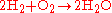Reaction stoichiometry describes the 2:1:2 ratio of hydrogen, oxygen, and water molecules in the above equation.

The term stoichiometry is also often used for the molar
Mole (unit)
The mole is a unit of measurement used in chemistry to express amounts of a chemical substance, defined as an amount of a substance that contains as many elementary entities as there are atoms in 12 grams of pure carbon-12 , the isotope of carbon with atomic weight 12. This corresponds to a value...

proportions of elements in stoichiometric compounds (composition stoichiometry). For example, the stoichiometry of hydrogen and oxygen in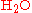is 2:1. In stoichiometric compounds, the molar proportions are whole numbers.

Stoichiometry is not only used to balance chemical equations but also used in conversions, i.e., converting from grams to moles, or from grams to millilitres. For example, to find the number of moles in 2.00 g of NaCl, one would do the following: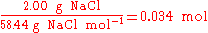In the above example, when written out in fraction form, the units of grams form a multiplicative identity, which is equivalent to one (g/g=1), with the resulting amount of moles (the unit that was needed), is shown in the following equation,Stoichiometry is also used to find the right amount of reactants to use in a chemical reaction
Chemical reaction
A chemical reaction is a process that leads to the transformation of one set of chemical substances to another. Chemical reactions can be either spontaneous, requiring no input of energy, or non-spontaneous, typically following the input of some type of energy, such as heat, light or electricity...

(stoichiometric amounts). An example is shown below using the thermite reaction,This equation shows that 1 mole of aluminium oxide and 2 moles of iron will be produced with 1 mole of and 2 moles of aluminium. So, to completely react with 85.0 g of (0.532 mol), 28.7 g (1.06 mol) of aluminium are needed.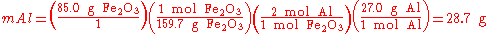## Different stoichiometries in competing reactions

Often, more than one reaction is possible given the same starting materials. The reactions may differ in their stoichiometry. For example, the methylation
Methylation
In the chemical sciences, methylation denotes the addition of a methyl group to a substrate or the substitution of an atom or group by a methyl group. Methylation is a form of alkylation with, to be specific, a methyl group, rather than a larger carbon chain, replacing a hydrogen atom...

of benzene
Benzene
Benzene is an organic chemical compound. It is composed of 6 carbon atoms in a ring, with 1 hydrogen atom attached to each carbon atom, with the molecular formula C6H6....

(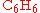), through a Friedel-Crafts reaction
Friedel-Crafts reaction
The Friedel–Crafts reactions are a set of reactions developed by Charles Friedel and James Crafts in 1877. There are two main types of Friedel–Crafts reactions: alkylation reactions and acylation reactions. This reaction type is a form of electrophilic aromatic substitution...

using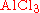as catalyst, may produce singly methylated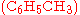, doubly methylated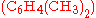, or still more highly methylated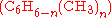products, as shown in the following example,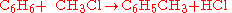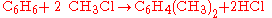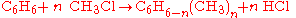In this example, which reaction takes place is controlled in part by the relative concentration
Concentration
In chemistry, concentration is defined as the abundance of a constituent divided by the total volume of a mixture. Four types can be distinguished: mass concentration, molar concentration, number concentration, and volume concentration...

s of the reactants.

## Stoichiometric coefficient

In layman's terms, the stoichiometric coefficient (or stoichiometric number in the IUPAC nomenclature) of any given component is the number of molecules which participate in the reaction as written.

For example, in the reaction CH4 + 2 O2 → CO2 + 2 H2O, the stoichiometric coefficient of CH4 would be 1 and the stoichiometric coefficient of O2 would be 2.

In more technically-precise terms, the stoichiometric coefficient in a chemical reaction
Chemical reaction
A chemical reaction is a process that leads to the transformation of one set of chemical substances to another. Chemical reactions can be either spontaneous, requiring no input of energy, or non-spontaneous, typically following the input of some type of energy, such as heat, light or electricity...

system
System
System is a set of interacting or interdependent components forming an integrated whole....

of the i–th component is defined as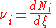orwhere Ni is the number of molecule
Molecule
A molecule is an electrically neutral group of at least two atoms held together by covalent chemical bonds. Molecules are distinguished from ions by their electrical charge...

s of i, and ξ is the progress variable or extent of reaction (Prigogine & Defay, p. 18; Prigogine, pp. 4–7; Guggenheim, p. 37 & 62).

The extent of reaction ξ can be regarded as a real (or hypothetical) product, one molecule of which is produced each time the reaction event occurs. It is the extensive quantity describing the progress of a chemical reaction equal to the number of chemical transformations, as indicated by the reaction equation on a molecular scale, divided by the Avogadro constant (it is essentially the amount of chemical transformations). The change in the extent of reaction is given by dξ = dnB/nB, where nB is the stoichiometric number of any reaction entity B (reactant or product) an dnB is the corresponding amount.

The stoichiometric coefficient νi represents the degree to which a chemical species participates in a reaction. The convention is to assign negative coefficients to reactants (which are consumed) and positive ones to products. However, any reaction may be viewed as "going" in the reverse direction, and all the coefficients then change sign (as does the free energy
Thermodynamic free energy
The thermodynamic free energy is the amount of work that a thermodynamic system can perform. The concept is useful in the thermodynamics of chemical or thermal processes in engineering and science. The free energy is the internal energy of a system less the amount of energy that cannot be used to...

). Whether a reaction actually will go in the arbitrarily-selected forward direction or not depends on the amounts of the substances
Chemical substance
In chemistry, a chemical substance is a form of matter that has constant chemical composition and characteristic properties. It cannot be separated into components by physical separation methods, i.e. without breaking chemical bonds. They can be solids, liquids or gases.Chemical substances are...

present at any given time, which determines the kinetics
Chemical kinetics
Chemical kinetics, also known as reaction kinetics, is the study of rates of chemical processes. Chemical kinetics includes investigations of how different experimental conditions can influence the speed of a chemical reaction and yield information about the reaction's mechanism and transition...

and thermodynamics
Thermodynamic equilibrium
In thermodynamics, a thermodynamic system is said to be in thermodynamic equilibrium when it is in thermal equilibrium, mechanical equilibrium, radiative equilibrium, and chemical equilibrium. The word equilibrium means a state of balance...

, i.e., whether equilibrium
Chemical equilibrium
In a chemical reaction, chemical equilibrium is the state in which the concentrations of the reactants and products have not yet changed with time. It occurs only in reversible reactions, and not in irreversible reactions. Usually, this state results when the forward reaction proceeds at the same...

lies to the right or the left.

If one contemplates actual reaction mechanism
Reaction mechanism
In chemistry, a reaction mechanism is the step by step sequence of elementary reactions by which overall chemical change occurs.Although only the net chemical change is directly observable for most chemical reactions, experiments can often be designed that suggest the possible sequence of steps in...

s, stoichiometric coefficients will always be integer
Integer
The integers are formed by the natural numbers together with the negatives of the non-zero natural numbers .They are known as Positive and Negative Integers respectively...

s, since elementary reactions always involve whole molecules. If one uses a composite representation of an "overall" reaction, some may be rational
Rational number
In mathematics, a rational number is any number that can be expressed as the quotient or fraction a/b of two integers, with the denominator b not equal to zero. Since b may be equal to 1, every integer is a rational number...

fractions
Fraction (mathematics)
A fraction represents a part of a whole or, more generally, any number of equal parts. When spoken in everyday English, we specify how many parts of a certain size there are, for example, one-half, five-eighths and three-quarters.A common or "vulgar" fraction, such as 1/2, 5/8, 3/4, etc., consists...

. There are often chemical species present that do not participate in a reaction; their stoichiometric coefficients are therefore zero. Any chemical species that is regenerated, such as a catalyst, also has a stoichiometric coefficient of zero.

The simplest possible case is an isomer
Isomer
In chemistry, isomers are compounds with the same molecular formula but different structural formulas. Isomers do not necessarily share similar properties, unless they also have the same functional groups. There are many different classes of isomers, like stereoisomers, enantiomers, geometrical...

ism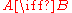in which νB = 1 since one molecule of B is produced each time the reaction occurs, while νA = −1 since one molecule of A is necessarily consumed. In any chemical reaction, not only is the total mass conserved
Conservation of mass
The law of conservation of mass, also known as the principle of mass/matter conservation, states that the mass of an isolated system will remain constant over time...

, but also the numbers of atom
Atom
The atom is a basic unit of matter that consists of a dense central nucleus surrounded by a cloud of negatively charged electrons. The atomic nucleus contains a mix of positively charged protons and electrically neutral neutrons...

s of each kind
Periodic table
The periodic table of the chemical elements is a tabular display of the 118 known chemical elements organized by selected properties of their atomic structures. Elements are presented by increasing atomic number, the number of protons in an atom's atomic nucleus...

are conserved, and this imposes corresponding constraints on possible values for the stoichiometric coefficients.

There are usually multiple reactions proceeding simultaneously in any natural
Nature
Nature, in the broadest sense, is equivalent to the natural world, physical world, or material world. "Nature" refers to the phenomena of the physical world, and also to life in general...

reaction system, including those in biology
Biology
Biology is a natural science concerned with the study of life and living organisms, including their structure, function, growth, origin, evolution, distribution, and taxonomy. Biology is a vast subject containing many subdivisions, topics, and disciplines...

. Since any chemical component can participate in several reactions simultaneously, the stoichiometric coefficient of the i–th component in the k–th reaction is defined as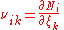so that the total (differential) change in the amount of the i–th component is.

Extents of reaction provide the clearest and most explicit way of representing compositional change, although they are not yet widely used.

With complex reaction systems, it is often useful to consider both the representation of a reaction system in terms of the amounts of the chemicals present { Ni } (state variables), and the representation in terms of the actual compositional degrees of freedom
Degrees of freedom (physics and chemistry)
A degree of freedom is an independent physical parameter, often called a dimension, in the formal description of the state of a physical system...

, as expressed by the extents of reaction { ξk }. The transformation from a vector
Vector space
A vector space is a mathematical structure formed by a collection of vectors: objects that may be added together and multiplied by numbers, called scalars in this context. Scalars are often taken to be real numbers, but one may also consider vector spaces with scalar multiplication by complex...

expressing the extents to a vector expressing the amounts uses a rectangular matrix
Matrix (mathematics)
In mathematics, a matrix is a rectangular array of numbers, symbols, or expressions. The individual items in a matrix are called its elements or entries. An example of a matrix with six elements isMatrices of the same size can be added or subtracted element by element...

whose elements are the stoichiometric coefficients [ νi k ].

The maximum and minimum for any ξk occur whenever the first of the reactants is depleted for the forward reaction; or the first of the "products" is depleted if the reaction as viewed as being pushed in the reverse direction. This is a purely kinematic
Kinematics
Kinematics is the branch of classical mechanics that describes the motion of bodies and systems without consideration of the forces that cause the motion....

restriction on the reaction simplex
Simplex
In geometry, a simplex is a generalization of the notion of a triangle or tetrahedron to arbitrary dimension. Specifically, an n-simplex is an n-dimensional polytope which is the convex hull of its n + 1 vertices. For example, a 2-simplex is a triangle, a 3-simplex is a tetrahedron,...

, a hyperplane
Hyperplane
A hyperplane is a concept in geometry. It is a generalization of the plane into a different number of dimensions.A hyperplane of an n-dimensional space is a flat subset with dimension n − 1...

in composition space, or N‑space, whose dimension
Dimension
In physics and mathematics, the dimension of a space or object is informally defined as the minimum number of coordinates needed to specify any point within it. Thus a line has a dimension of one because only one coordinate is needed to specify a point on it...

ality equals the number of linearly-independent
Linear independence
In linear algebra, a family of vectors is linearly independent if none of them can be written as a linear combination of finitely many other vectors in the collection. A family of vectors which is not linearly independent is called linearly dependent...

chemical reactions. This is necessarily less than the number of chemical components, since each reaction manifests a relation between at least two chemicals. The accessible region of the hyperplane depends on the amounts of each chemical species actually present, a contingent fact. Different such amounts can even generate different hyperplanes, all of which share the same algebraic stoichiometry.

In accord with the principles of chemical kinetics
Chemical kinetics
Chemical kinetics, also known as reaction kinetics, is the study of rates of chemical processes. Chemical kinetics includes investigations of how different experimental conditions can influence the speed of a chemical reaction and yield information about the reaction's mechanism and transition...

and thermodynamic equilibrium
Thermodynamic equilibrium
In thermodynamics, a thermodynamic system is said to be in thermodynamic equilibrium when it is in thermal equilibrium, mechanical equilibrium, radiative equilibrium, and chemical equilibrium. The word equilibrium means a state of balance...

, every chemical reaction is reversible, at least to some degree, so that each equilibrium point must be an interior point
Interior (topology)
In mathematics, specifically in topology, the interior of a set S of points of a topological space consists of all points of S that do not belong to the boundary of S. A point that is in the interior of S is an interior point of S....

of the simplex. As a consequence, extrema for the ξ's will not occur unless an experimental system is prepared with zero initial amounts of some products.

The number of physically-independent reactions can be even greater than the number of chemical components, and depends on the various reaction mechanisms. For example, there may be two (or more) reaction paths for the isomerism above. The reaction may occur by itself, but faster and with different intermediates, in the presence of a catalyst.

The (dimensionless) "units" may be taken to be molecule
Molecule
A molecule is an electrically neutral group of at least two atoms held together by covalent chemical bonds. Molecules are distinguished from ions by their electrical charge...

s or moles
Mole (unit)
The mole is a unit of measurement used in chemistry to express amounts of a chemical substance, defined as an amount of a substance that contains as many elementary entities as there are atoms in 12 grams of pure carbon-12 , the isotope of carbon with atomic weight 12. This corresponds to a value...

. Moles are most commonly used, but it is more suggestive to picture incremental chemical reactions in terms of molecules. The Ns and ξ's are reduced to molar units by dividing by Avogadro's number
Avogadro's number
In chemistry and physics, the Avogadro constant is defined as the ratio of the number of constituent particles N in a sample to the amount of substance n through the relationship NA = N/n. Thus, it is the proportionality factor that relates the molar mass of an entity, i.e...

. While dimensional mass
Mass
Mass can be defined as a quantitive measure of the resistance an object has to change in its velocity.In physics, mass commonly refers to any of the following three properties of matter, which have been shown experimentally to be equivalent:...

units may be used, the comments about integers are then no longer applicable.

## Stoichiometry matrix

In complex reactions, stoichiometries are often represented in a more compact form called the stoichiometry matrix. The stoichiometry matrix is denoted by the symbol,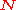.

If a reaction network hasreactions andparticipating molecular species then the stoichiometry matrix will have correspondingrows andcolumns.

For example, consider the system of reactions shown below:
S1 → S2

5S3 + S2 → 4S3 + 2S2

S3 → S4

S4 → S5.

This systems comprises four reactions and five different molecular species. The stoichiometry matrix for this system can be written as: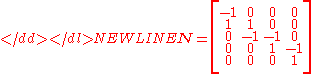where the rows correspond to S1, S2, S3, S4 and S5, respectively. Note that the process of converting a reaction scheme into a stoichiometry matrix can be a lossy transformation, for example, the stoichiometries in the second reaction simplify when included in the matrix. This means that it is not always possible to recover the original reaction scheme from a stoichiometry matrix.

Often the stoichiometry matrix is combined with the rate vector, v to form a compact equation describing the rates of change of the molecular species: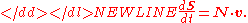## Gas stoichiometry

Gas stoichiometry is the quantitative relationship (ratio) between reactants and products in a chemical reaction
Chemical reaction
A chemical reaction is a process that leads to the transformation of one set of chemical substances to another. Chemical reactions can be either spontaneous, requiring no input of energy, or non-spontaneous, typically following the input of some type of energy, such as heat, light or electricity...

with reactions that produce gases. Gas stoichiometry applies when the gases produced are assumed to be ideal
Ideal gas
An ideal gas is a theoretical gas composed of a set of randomly-moving, non-interacting point particles. The ideal gas concept is useful because it obeys the ideal gas law, a simplified equation of state, and is amenable to analysis under statistical mechanics.At normal conditions such as...

, and the temperature, pressure, and volume of the gases are all known. The ideal gas law is used for these calculations. Often, but not always, the standard temperature and pressure (STP) are taken as 0°C and 1 bar and used as the conditions for gas stoichiometric calculations.

Gas stoichiometry calculations solve for the unknown volume
Volume
Volume is the quantity of three-dimensional space enclosed by some closed boundary, for example, the space that a substance or shape occupies or contains....

or mass
Mass
Mass can be defined as a quantitive measure of the resistance an object has to change in its velocity.In physics, mass commonly refers to any of the following three properties of matter, which have been shown experimentally to be equivalent:...

of a gaseous product or reactant. For example, if we wanted to calculate the volume of gaseous NO2 produced from the combustion of 100 g of NH3, by the reaction:
4NH3 (g) + 7O2 (g) → 4NO2 (g) + 6H2O (l)

we would carry out the following calculations:There is a 1:1 molar ratio of NH3 to NO2 in the above balanced combustion reaction, so 5.871 mol of NO2 will be formed. We will employ the ideal gas law
Ideal gas law
The ideal gas law is the equation of state of a hypothetical ideal gas. It is a good approximation to the behavior of many gases under many conditions, although it has several limitations. It was first stated by Émile Clapeyron in 1834 as a combination of Boyle's law and Charles's law...

to solve for the volume at 0 °C (273.15 K) and 1 atmosphere using the gas law constant
Gas constant
The gas constant is a physical constant which is featured in many fundamental equations in the physical sciences, such as the ideal gas law and the Nernst equation. It is equivalent to the Boltzmann constant, but expressed in units of energy The gas constant (also known as the molar, universal,...

of R = 0.08206 L · atm · K−1 · mol−1 :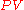Gas stoichiometry often involves having to know the molar mass
Molar mass
Molar mass, symbol M, is a physical property of a given substance , namely its mass per amount of substance. The base SI unit for mass is the kilogram and that for amount of substance is the mole. Thus, the derived unit for molar mass is kg/mol...

of a gas, given the density
Density
The mass density or density of a material is defined as its mass per unit volume. The symbol most often used for density is ρ . In some cases , density is also defined as its weight per unit volume; although, this quantity is more properly called specific weight...

of that gas. The ideal gas law can be re-arranged to obtain a relation between the density
Density
The mass density or density of a material is defined as its mass per unit volume. The symbol most often used for density is ρ . In some cases , density is also defined as its weight per unit volume; although, this quantity is more properly called specific weight...

and the molar mass
Molar mass
Molar mass, symbol M, is a physical property of a given substance , namely its mass per amount of substance. The base SI unit for mass is the kilogram and that for amount of substance is the mole. Thus, the derived unit for molar mass is kg/mol...

of an ideal gas:and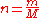and thus: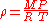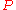where: = absolute gas pressurePressurePressure is the force per unit area applied in a direction perpendicular to the surface of an object. Gauge pressure is the pressure relative to the local atmospheric or ambient pressure.- Definition :... = gas volumeVolumeVolume is the quantity of three-dimensional space enclosed by some closed boundary, for example, the space that a substance or shape occupies or contains.... = number of molesMole (unit)The mole is a unit of measurement used in chemistry to express amounts of a chemical substance, defined as an amount of a substance that contains as many elementary entities as there are atoms in 12 grams of pure carbon-12 , the isotope of carbon with atomic weight 12. This corresponds to a value... = universal ideal gas law constant = absolute gas temperatureTemperatureTemperature is a physical property of matter that quantitatively expresses the common notions of hot and cold. Objects of low temperature are cold, while various degrees of higher temperatures are referred to as warm or hot... = gas density at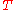and= mass of gas = molar mass of gas

## Stoichiometric air-fuel ratios of common fuels

Fuel By mass By volume Percent fuel by mass
Gasoline 14.6 : 1 6.8%
Natural gas 14.5 : 1 9.7 : 1 5.8%
Propane (LP) 15.67 : 1 23.9 : 1 6.45%
Ethanol 9 : 1 11.1%
Methanol 6.47 : 1 15.6%
Hydrogen 34.3 : 1 2.39 : 1 2.9%
Diesel 14.5 : 1 0.094 : 1 6.8%

Gasoline engines can run at stoichiometric air-to-fuel ratio, because gasoline is quite volatile and is mixed (sprayed or carburetted) with the air prior to ignition. Diesel engines, in contrast, run lean, with more air available than simple stoichiometry would require. Diesel fuel is less volatile and is effectively burned as it is injected, leaving less time for evaporation and mixing. Thus, it would form soot (black smoke) at stoichiometric ratio.

## External links

The source of this article is wikipedia, the free encyclopedia.  The text of this article is licensed under the GFDL.